Contact us

# The Math Behind Android Wear 2.0

With the advent of Android Wear 2.0 and the new features it brings to the table — a standalone Play store and the Complications API, to name exactly two — Google hopes to put more smartwatches on presently dumb wrists. This endeavor requires Wear developers, novice and seasoned alike, creating 2.0-targeted apps and eye-catching watch faces. To help get these movers and shakers moving and shaking, Google has provided code lessons in its developers portal as well as an example watch face template in Android Studio in addition to the many watch face tutorials already online. With such a vast wealth of resources available to help you publish an Android watch face, why do you need this article?

Well, you don’t. You can readily compile and run Google’s watch face template and see a working example on your emulator, taking for granted the math code already written for you with which to plug and play your own bitmaps and renders. But you’re a pedant. You’re mentally and emotionally unable to ignore lines of code that you don’t understand. And you know that if you want to take full advantage of a Wear canvas, you’ll eventually have to look that code in the eye and tell it you’re the boss around here. Other resources skip over explaining the math portion of watch face development. This post fills in those gaps.

## Back To School

The circle. Just what is a circle? No one knows. But it looks eerily similar to the surface of many a watch face — not a coincidence, as new smartwatch players like Michael Kors and Fossil seem to be putting more of the rounded displays on the market instead of square ones. So capitalism demands we understand a few things about the circle if we’re to translate its magic into code.Embedded content: https://www.youtube.com/watch?v=4J9MRYJz9-4

Picture a circular watch in your head. Or maybe you’re wearing one right now. Take a look at it. Don’t be scared. Are there the numbers 1 through 12? Are they the same distance from each other? Perhaps there’s a hand that indicates the current time in seconds. Does it appear to move the same distance every second? But these distances are different from one where you walk a straight line from where you are to the door. These distances are measured by points along circle.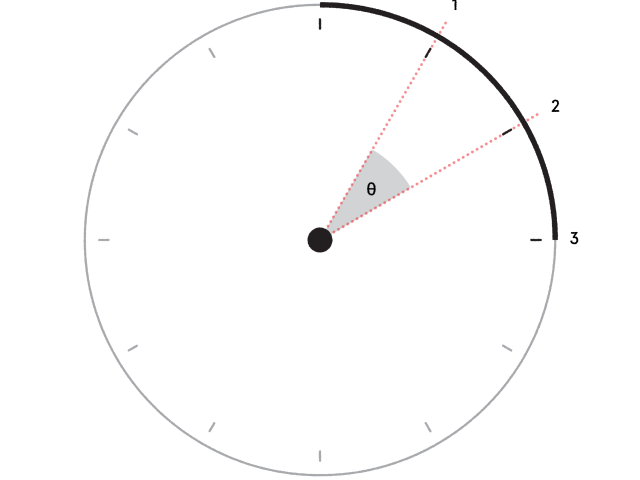Picture another circular watch twice the size as the first. We could choose to notice that the distance between the 1 and the 2 has doubled. But imagine a line from the center of the watch to the 1. Then imagine another line from the center to the 2. That angle (the symbol θ, called “theta”) between the two lines? It’s exactly the same, whether you’re looking at the small watch or the one twice as large. If we just work with that angle and not even care about the distance between points on a circle, we have a simple foundation for ticking a hand between hours or minutes or seconds no matter the size of the watch.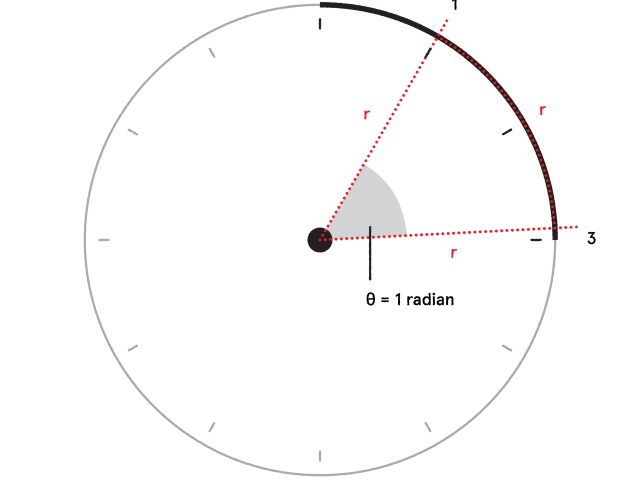So how about those lines from the center to a point on the circle? That’s the radius. Now imagine a distance along the circle (r in the diagram above) that’s the same length as the radius (also r, since they’re equal). Or rather, imagine if the distance traveled to get from 1 to 2 is the same length as the line from the center to 1. The angle created in that situation is measured as one radian. How many of these radians would you need if you wanted to travel the total length of a circle? You could draw it out by yourself, you curious cat, or you could just believe mathematicians when they tell you that you would need exactly 2 * π radians, where π is a number close to 3.14, as you hopefully remember. This all means that the angle in radians between 12 o’clock and 3 o’clock is exactly π / 2; between 12 o’clock and 6 o’clock exactly π; between 12 o’clock and 9 o’clock exactly (3 * π) / 2; and the angle of a complete rotation is 2π radians.

Another thing you may remember: right triangles! Recall that a right triangle is a special triangle where one angle measures 90 degrees. Why do we care about this? Let’s look again at those lines pointing from the center to one of the hours on a watch face. What if we gave those lines a reason for being? What if we gave those lines purpose? What if those lines were the hour, minute, and second hands? Useful! And metaphysical. Gottfried Leibniz would be proud.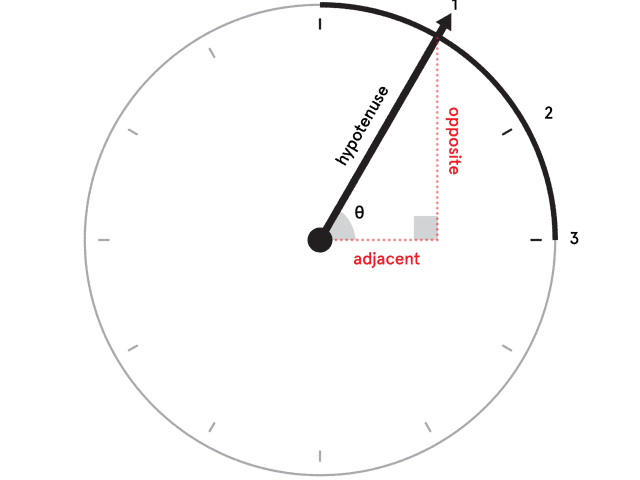Let’s take an hour hand that points to 1 o’clock. You can see that a right triangle can be formed with one side on an imaginary x-axis and another parallel to a y-axis. The hypotenuse is then the length of the hand. The endpoint of the hand can be described by an (x, y) ordered pair comprising of its position along the x-axis and its position along the y-axis.

How do we get these x and y values? Do you remember SOHCAHTOA? That mnemonic abomination helps us vaguely remember that sin(θ) = opposite / hypotenuse and cos(θ) = adjacent / hypotenuse. So if we know the length of the hour hand (the hypotenuse), we also know that the length of the opposite side is sin(θ) * hypotenuse and that the length of the adjacent side is cos(θ) * hypotenuse. The (x, y) pair of the endpoint is then just (length of adjacent, length of opposite). Remember these equations, as they will come in handy once you look at how Android draws bitmaps on a Canvas object. Which leads us finally to…

## Watch Face Java

Let’s take the actual code examples Google gives us and decipher the math they don’t bother to explain.

First up, if you’ve been to the Google Developers Drawing Watch Faces lesson, in the example onDraw() method you may have seen this:

``// Compute rotations and lengths for the clock hands.float seconds = mCalendar.get(Calendar.SECOND) + mCalendar.get(Calendar.MILLISECOND) / 1000f;float secRot = seconds / 60f * TWO_PI;float minutes = mCalendar.get(Calendar.MINUTE) + seconds / 60f;float minRot = minutes / 60f * TWO_PI;float hours = mCalendar.get(Calendar.HOUR) + minutes / 60f;float hrRot = hours / 12f * TWO_PI;``

So to draw the second hand, you first need to get its angle of rotation, designated by the variable secRot in this example. Finding the rotation is simply a matter of finding how much of a pizza pie you want to eat, where our pizza is cut up into an insane 60 slices. Pointing to 4 o’clock? Our current time must be 20 seconds, or 20 slices. So 20 / 60, or a ratio of 1 / 3. But one-third of what? The population of Newfoundland? Close. One-third the angle of rotating in a complete circle! So multiply 1 / 3 by 2π and we have our rotation in radians if we want our second hand to point towards 4 o’clock.

It’s nearly the same deal for our minute and hour hands, but if you look closely at the variable called minutes, you’ll see that it’s not simply based on the current time in minutes. There’s also some fraction dealing with the current time in seconds. This extra code is needed because the minute and hour hands on a watch face don’t actually point exactly at the current minute and hour unless it’s that exact time. If the time were, say, 6:30:30, the hour hand would point halfway between 6 and 7, and the minute hand halfway between 30 and 31, since we’re at 30 seconds. You can involve milliseconds if you want the second hand to smoothly sweep around the circle, or ignore them if you just need hard ticks.

``float secX = (float) Math.sin(secRot) * secLength;float secY = (float) –Math.cos(secRot) * secLength;canvas.drawLine(centerX, centerY, centerX + secX, centerY + secY, mSecondPaint);``

Now that we have our angle of rotation for the second hand, we can draw the bad boy. What information do we need to draw a bad boy? On a sheet of graph paper, we just need the starting point and the ending point, where each point is an (x, y) ordered pair. Android’s drawLine() method (as seen above) requires the starting point along the x-axis, the starting point along the y-axis, the x-axis endpoint, the y-axis endpoint, and a Paint object (if we want one). Finally, our SOHCAHTOA comes into play. We take the variable secLength as the hypotenuse of the second hand, and then we recall from the previous section that cos(secRot) * secLength gets us the x-coordinate and sin(secRot) * secLength the y-coordinate, exactly like the example abo— WAIT A MINUTE. WHAT. THAT’S NOT WHAT IT SAYS AT ALL. MATH IS A LIE. SOYLENT GREEN IS PEOPLE.

Why is Android saying that our x-coordinate is sin(θ) * length and our y-coordinate is some sort of negative number nonsense when it was clearly and eloquently established in the section prior how to arrive at these calculations? Well, the exercise we did before involved a Cartesian coordinate system where the the x-axis points towards 3 o’clock and the y-axis towards 12 o’clock. But in such a coordinate plane, if our angle of rotation is zero, then we’re drawing a line right on top of the x-axis! In such a coordinate plane, if we have angles of rotations that we expect to point at 12:00:00, in actuality, all our hands would point at 3 o’clock!

For watch faces, our angles of rotation need to be calculated with respect to an axis pointing towards 12 o’clock. This variation means that whatever value of θ we want needs to be translated counterclockwise around the plane by 90 degrees so that we can keep our mindset in terms of how a watch looks and behaves (our starting point is 12 o’clock and NOT 3 thank you very much). Thankfully, mathematicians have already determined a set of trigonometric identities to help us with our dilemma. Sly dogs, they.

First, recall the original bad boys to get the (x, y) ordered pair of the endpoint of a line:

``x = cos(θ) * hypotenuse y = sin(θ) * hypotenuse ``

For subtracting an angle by a certain value, we can use the trigonometric identities:

``cos(α – β) = cos(α) * cos(β) + sin(α) * sin(β)sin(α – β) = sin(α) * cos(β) – cos(α) * sin(β) We want to subtract by 90 degrees, so we then have: cos(θ – 90°) = cos(θ) * cos(90°) + sin(θ) * sin(90°) sin(θ – 90°) = sin(θ) * cos(90°) – cos(θ) * sin(90°) ``

Hopefully you also remember your sine and cosine curves? If you do, you know that at 90°, the value of the cosine function is 0 and the value of the sine function is 1. Plugging in these bad boys gets us:

``cos(θ – 90°) = cos(θ) * 0 + sin(θ) * 1 = sin(θ) sin(θ – 90°) = sin(θ) * 0 – cos(θ) * 1 = –cos(θ) ``

Which is precisely the equation set that Google gives us in their code examples:

``xTranslated = cos(θ – 90°) * hypotenuse = sin(θ) * hypotenuse yTranslated = sin(θ – 90°) * hypotenuse = –cos(θ) * hypotenuse``

## Knowledge++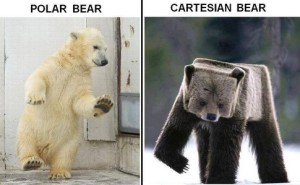Well, now you know how it all goes down. With this basic foundation, you can math your way into any watch face you can imagine. Maybe you want to animate tracing out some hella dope, hella arbitrary shape. If you already have the dope shape’s dope equation in polar form (i.e. you see angles and sines and cosines), terrific! You should then be able to identify x and y positions at any given moment in time (based on your secRot, minRot, and hrRot variables, if you’re following Google’s practice as shown previously). However, if your equation involves Cartesian coordinates (i.e. strictly based on x and y positions), you can convert it to a polar one so you can continue to think in terms of angles of rotation, watch face style.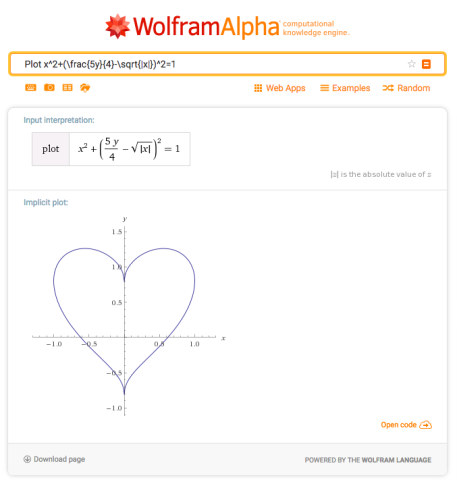But it doesn’t end there! Here are all the cool things you can do now that you know watch face math:

1. Make watch faces
2. That’s it

So get out there and be not fun at parties!